1. 游戏介绍

0x1：游戏规则

1. 由老板发出第一个种子红包，金额 b = 500，红包分成100份，每份金额是随机的，红包发到钉钉群后，大家可以由两种选择：抢 or 不抢；
2. 如果选择不抢，则本轮无损失也没有收益。
3. 如果选择了抢，还需要拼手速，因为大部分时候选择抢的同学个数依然大于红包最大拆分份数；
4. 所有红包拆分份数都被抢完之后，由本轮抢的最大金额的同学发下轮的红包，每轮的红包金额需要在之前的基础上增加200；
5. 游戏循环进行，直到达到5000元上限结束；

0x2：游戏策略分析

1. 伯努利实验抽象建模

p=0.99：不是抢到最大的那份红包；
p=0.01：抢到最大的那份红包；

2. 基于数学期望分析游戏的损失风险

（抢到非最大那份红包的概率 * 收益 - 抢到最大那份红包的概率 * 损失 ）* 本轮的红包金额 = （99 / 100 * 1 / 100 - 1 / 100）* （500 + 200n）= 1 / 10000 * （500 + 200n）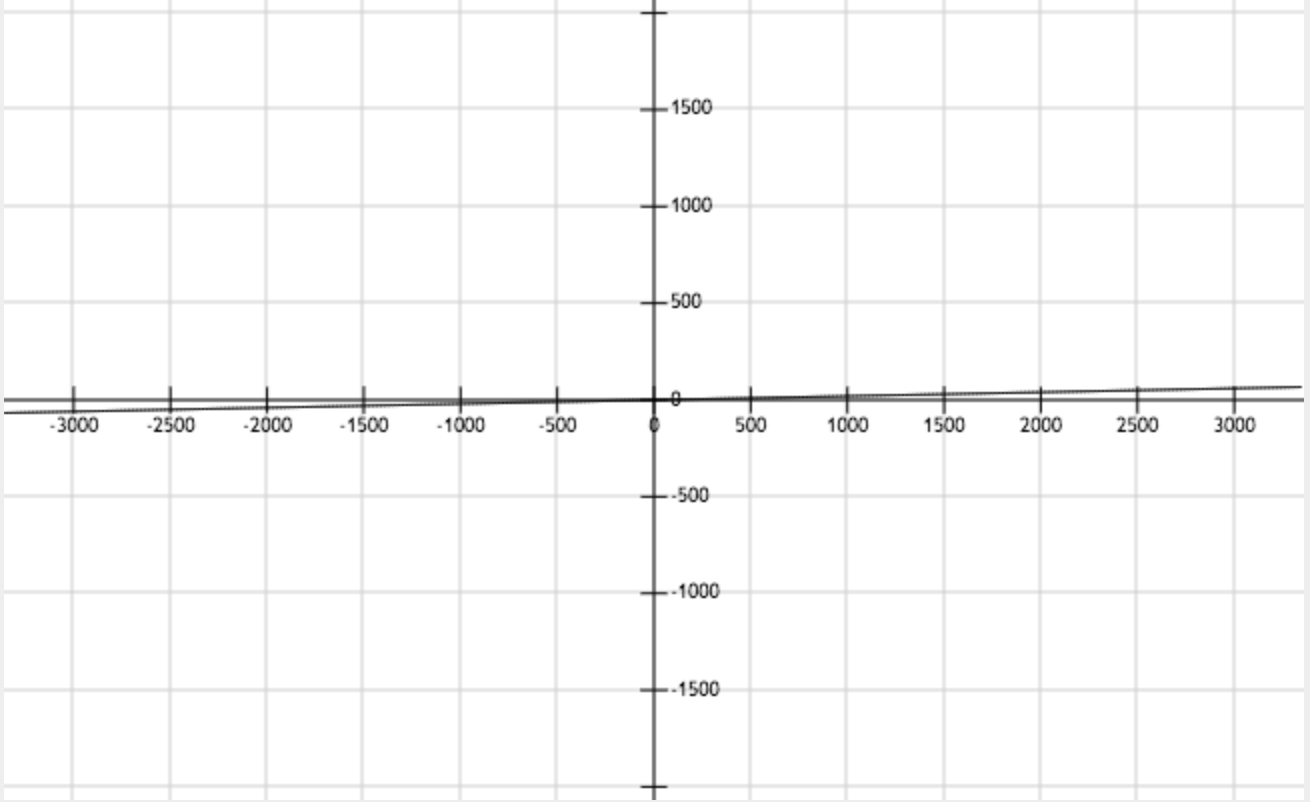3. 基于几何分布的角度分析游戏的损失风险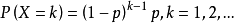p = 1/100：出现负结果，即抽中最大红包；
k=随机变量，表示参与游戏的次数；

P（X = k）=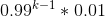，k=1，2，3...N.

4. 基于泊松分布的角度分析游戏的收益风险

1. n：游戏的次数；
2. p=99/100：盈利的概率；
3. λ = np = 0.99n：泊松分布参数；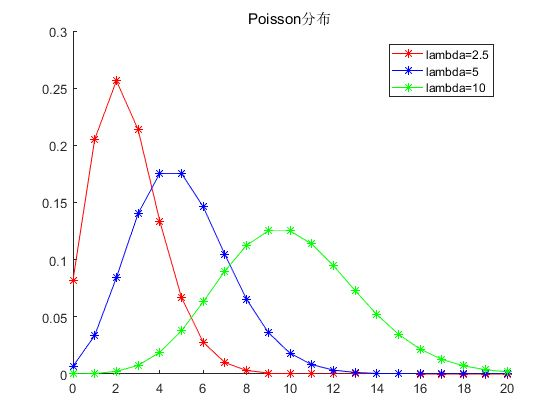0x4：画出泊松分布累计概率分布图

# -*- coding:utf-8 -*-

from scipy.stats import poisson
import matplotlib as mpl
import matplotlib.pyplot as plt
import numpy as np

N = 20
p = 0.99
# mu = N * p
data = poisson.rvs(mu=p*N, loc=0, size=N)
print "Mean: %f" % np.mean(data)
print "SD: %f" % np.std(data, ddof=1)

plt.figure()
plt.hist(data, bins=1, normed=True, histtype='stepfilled')
plt.xlim(0, N * 1)
plt.xlabel("Rounds")
plt.title("Rea Packge Game")
plt.show()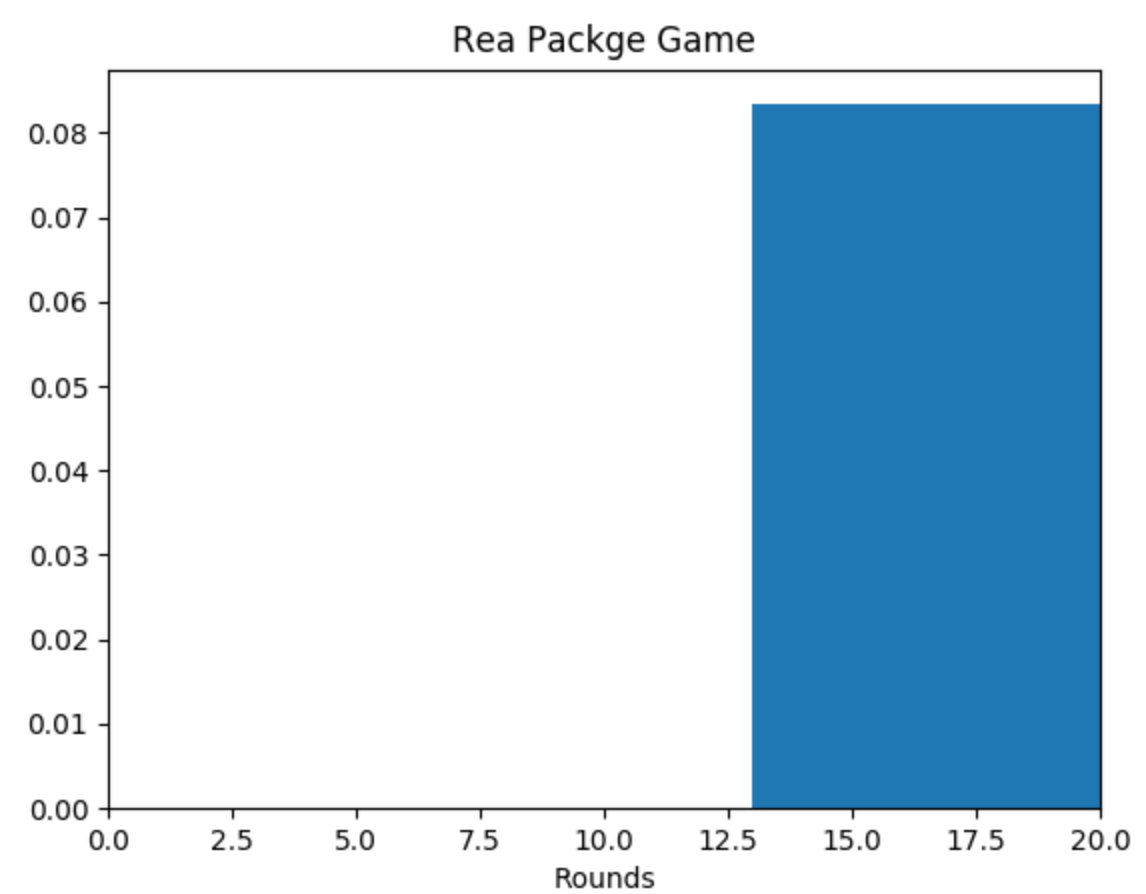1. P（参与了13次，一次都没有抽中最大红包）= 0.99^13 = 0.877521022999：概率还是相比比较高的；
2. E（损失）=  1 / 10000 * （500 + 200 * 13）= 0.31

2. 红包游戏该怎么玩呢？

0x1：最终的策略

1. 首先，根据大老板给出的种子金额以及封顶金额进行一个估算，得到 N 的最大值，例如本文的20；
2. 然后，计算【0，N】之间的95%正态区间的负区间，也就是左区间的整数MIN值，例如本文的13；
3. 将13作为我们整晚游戏的参与上限，同时建立止损机制，如果在13轮内都没有抽中最大红包，则继续，但是最多只参与13次，如果在这之前不小心抽总了一次最大红包，立即停止，及时止损；

that's it!!

posted @ 2019-02-24 19:58 郑瀚Andrew.Hann 阅读(...) 评论(...) 编辑 收藏